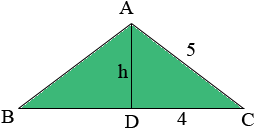Subject: height of a triangle Name: Jeni Who are you: Other I need to know how to figure out the height of a triangle when all I know are the sides and base. The sides are 5 and the base is 8 What do I do? Please help:) Hi Jeni, The fact that you have an isosceles triangle (two sides have the same length) makes this question easier that it might otherwise be. I drew your triangle ABC and joined the midpoint of the base D to the opposite vertex.Triangles ABD and ADC are congruent and hence it follows that angle ADC is a right angle. Hence triangle ADB s a right triangle and thus by Pythagoras' Theorem 52 = 42 + h2 Solve for h. Penny